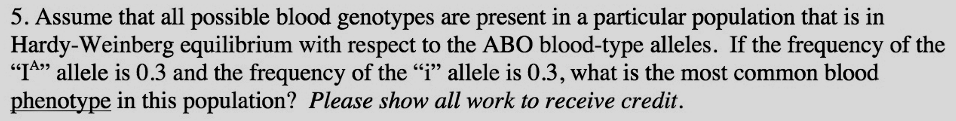# Problem: Assume that all possible blood genotypes are present in a particular population that is in Hardy-Weinberg equilibrium with respect to the ABO blood-type alleles. If the frequency of the "IA" allele is 0.3 and the frequency of the "i" allele if 0.3, what is the most common blood phenotype in this population?

###### FREE Expert Solution

In a system that is at Hardy-Weinberg equilibrium, the frequency of alleles can lead to a good estimation of the phenotype frequency of the gene in question, or it may be the other way around where the allele frequency can be computed from the available phenotypic frequencies. Note that gene frequencies are usually expressed in percentage. The given problem is known to use three alleles: one for blood type A, another for B, and lastly for O.###### Problem Details

Assume that all possible blood genotypes are present in a particular population that is in Hardy-Weinberg equilibrium with respect to the ABO blood-type alleles. If the frequency of the "IA" allele is 0.3 and the frequency of the "i" allele if 0.3, what is the most common blood phenotype in this population?